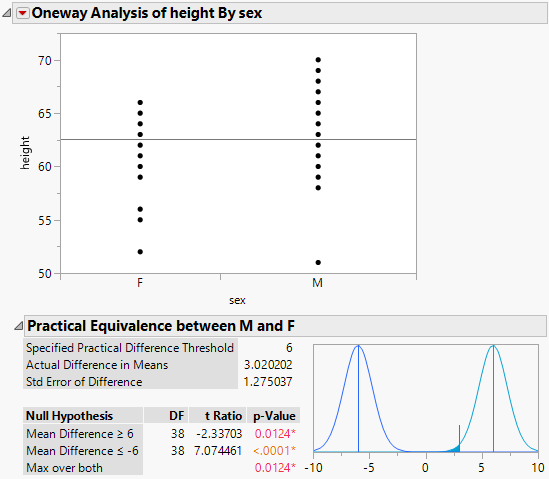Publication date: 08/13/2020

## Example of an Equivalence Test

This example uses the Big Class.jmp sample data table. Examine if the difference in height between males and females is less than 6 inches.

1. Select Help > Sample Data Library and open Big Class.jmp.

2. Select Analyze > Fit Y by X.

3. Select height and click Y, Response.

4. Select sex and click X, Factor.

5. Click OK.

6. Click the red triangle Oneway Analysis of height By sex and select Equivalence Test.

7. Type 6 as the difference considered practically zero.

8. Click OK.

Figure 6.30 Example of an Equivalence TestUsing two one-sided tests, you can see that the p-value is small for both. Therefore, you can conclude that the difference in population means is located somewhere between -6 and 6. For your purposes, you can declare the means to be practically equivalent.